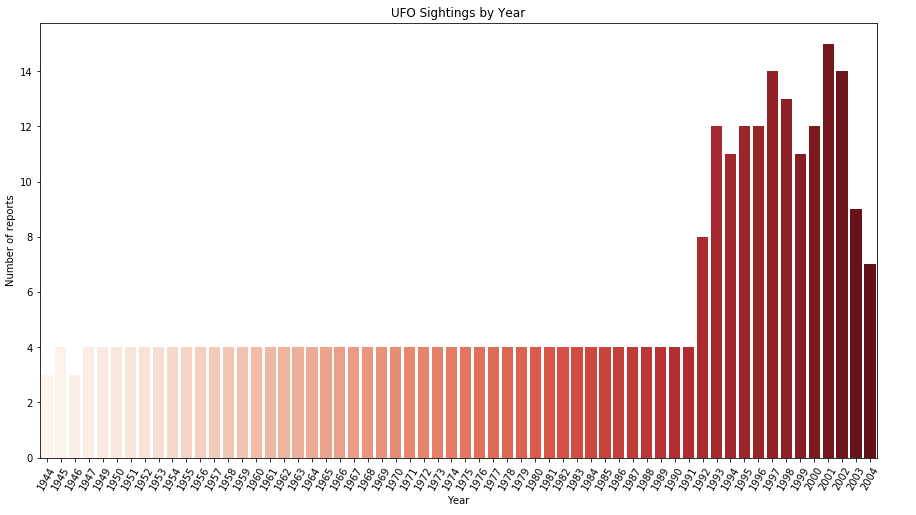﻿ Pandas Datetime: Create a graphical analysis of UFO Sightings year - w3resource# Pandas Datetime: Create a graphical analysis of UFO Sightings year

## Pandas Datetime: Exercise-18 with Solution

Write a Pandas program to create a graphical analysis of UFO (unidentified flying object) Sightings year.

Sample Solution:

Python Code:

``````import pandas as pd
import matplotlib.pyplot as plt
import seaborn as sns
df['Date_time'] = df['Date_time'].astype('datetime64[ns]')
df["ufo_yr"] = df.Date_time.dt.year
years_data = df.ufo_yr.value_counts()
years_index = years_data.index  # x ticks
years_values = years_data.get_values()
plt.figure(figsize=(15,8))
plt.xticks(rotation = 60)
plt.title('UFO Sightings by Year')
plt.xlabel("Year")
plt.ylabel("Number of reports")
years_plot = sns.barplot(x=years_index[:60],y=years_values[:60], palette = "Reds")
``````

Sample Output:

``````

Python Code Editor:

Have another way to solve this solution? Contribute your code (and comments) through Disqus.

What is the difficulty level of this exercise?

﻿

## Python: Tips of the Day

Checks if the given number falls within the given range.

Example:

```def tips_range(n, start, end = 0):
return start <= n <= end if end >= start else end <= n <= start
print(tips_range(2, 4, 6))
print(tips_range(4, 8))
print(tips_range(1, 3, 5))
print(tips_range(1, 3))
```

Output:

```False
True
False
True
```